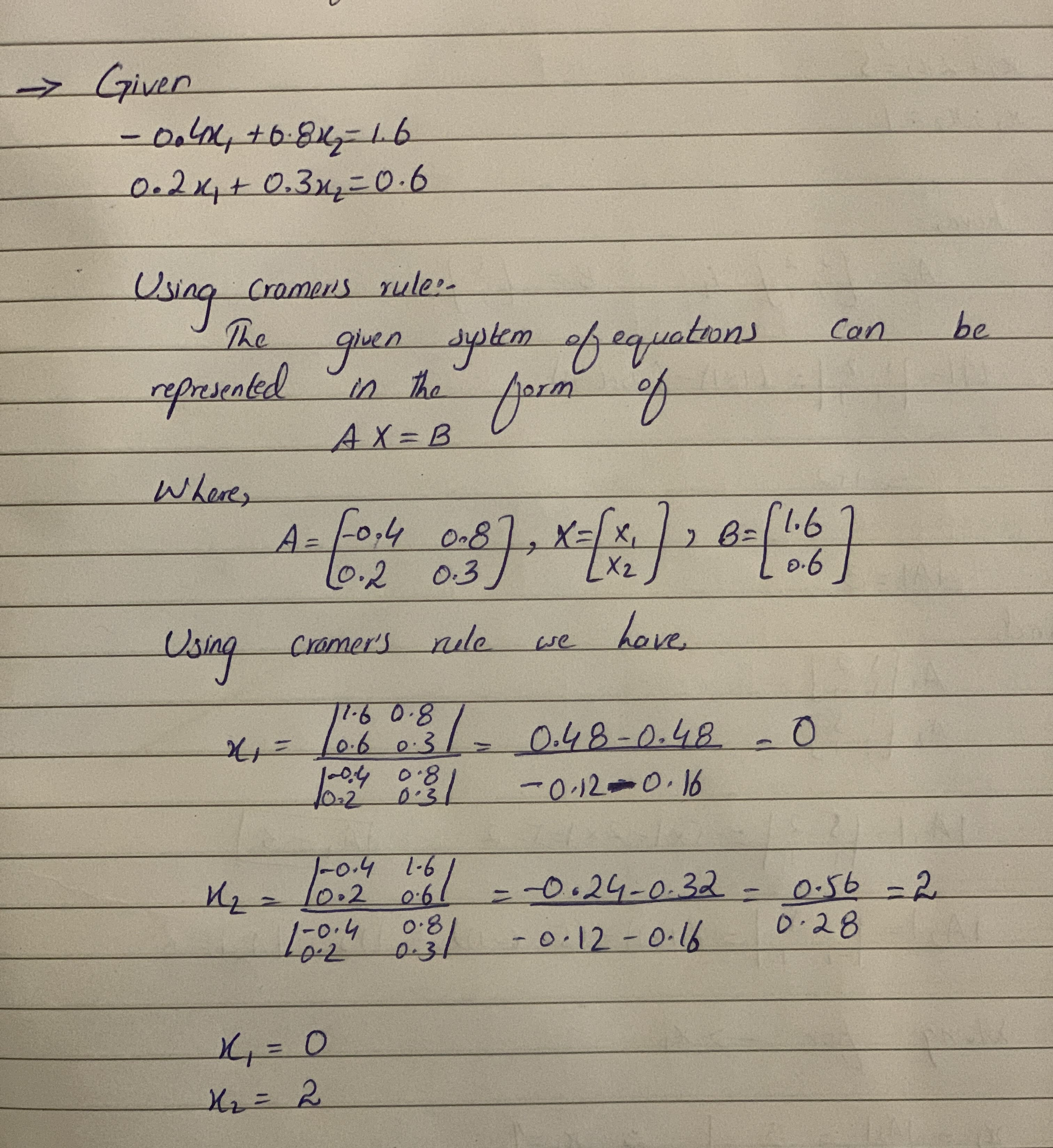# Question# Use cramer's rule to solve -0.4x_1+0.8x_2=1.6 0.2x_1+0.3x_2=0.6

Forms of linear equations
ANSWEREDUse cramer's rule to solve
$$-0.4x_1+0.8x_2=1.6$$
$$0.2x_1+0.3x_2=0.6$$